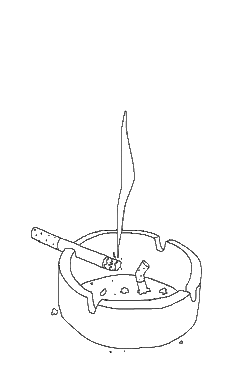# June 2020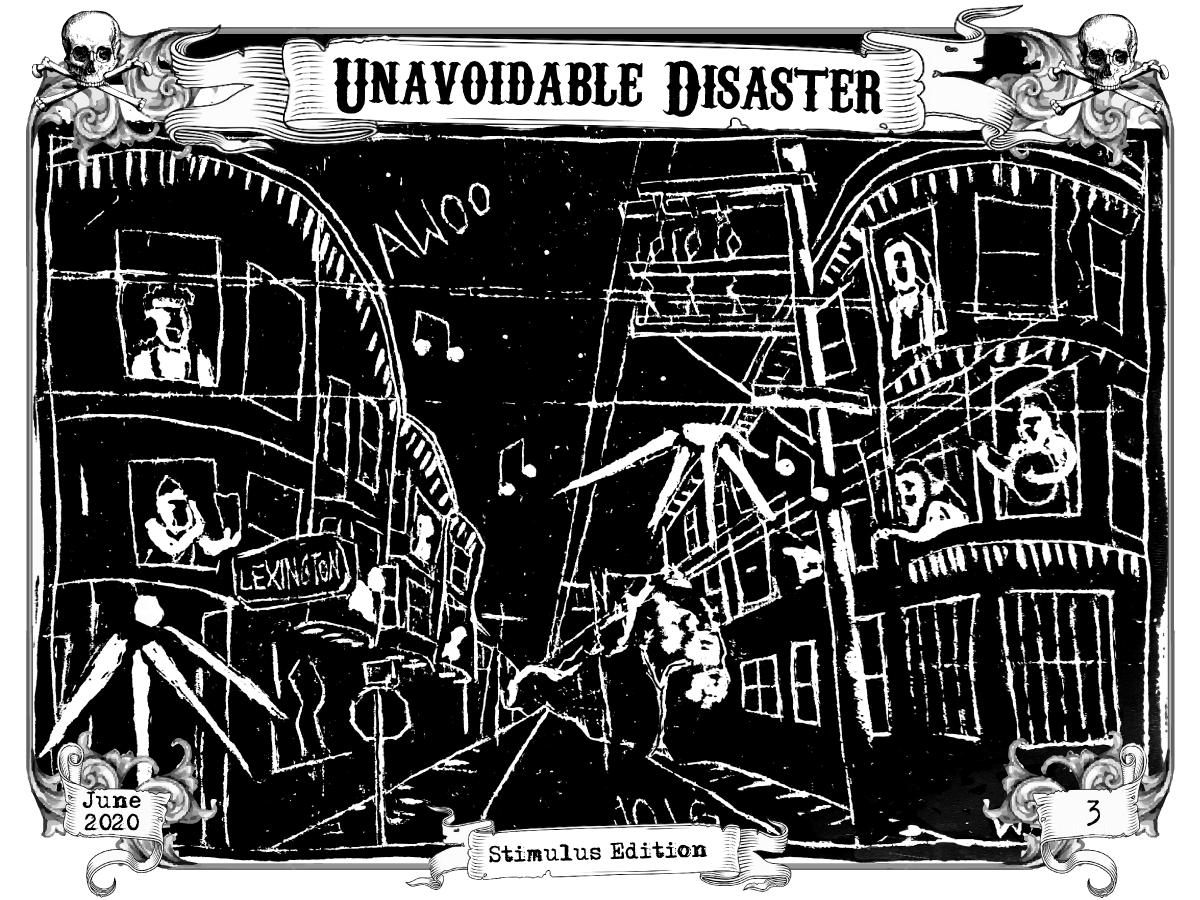x

Page 1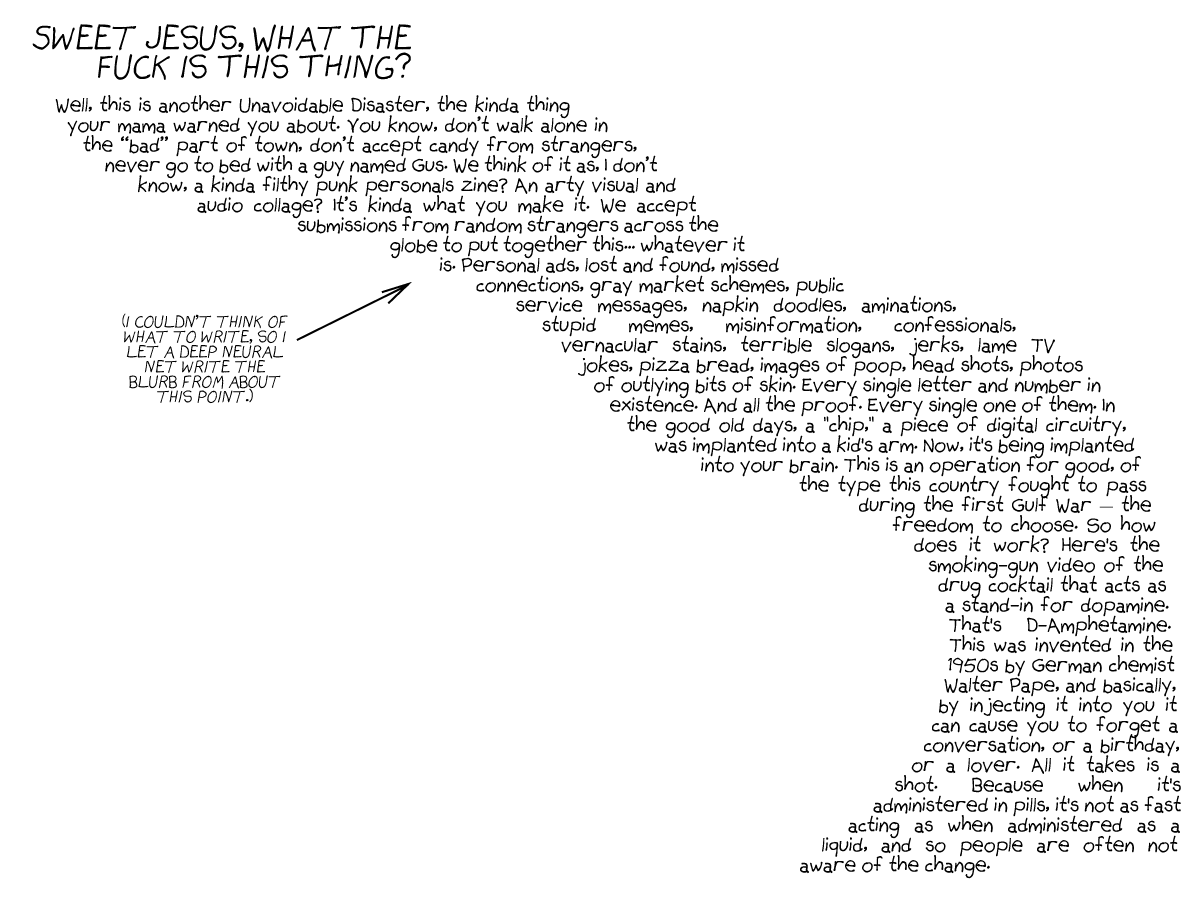xxxx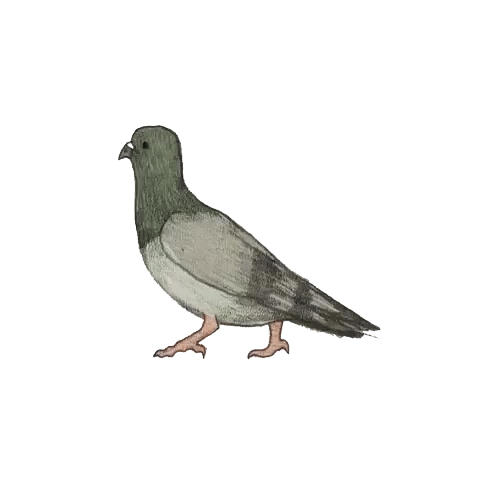xxx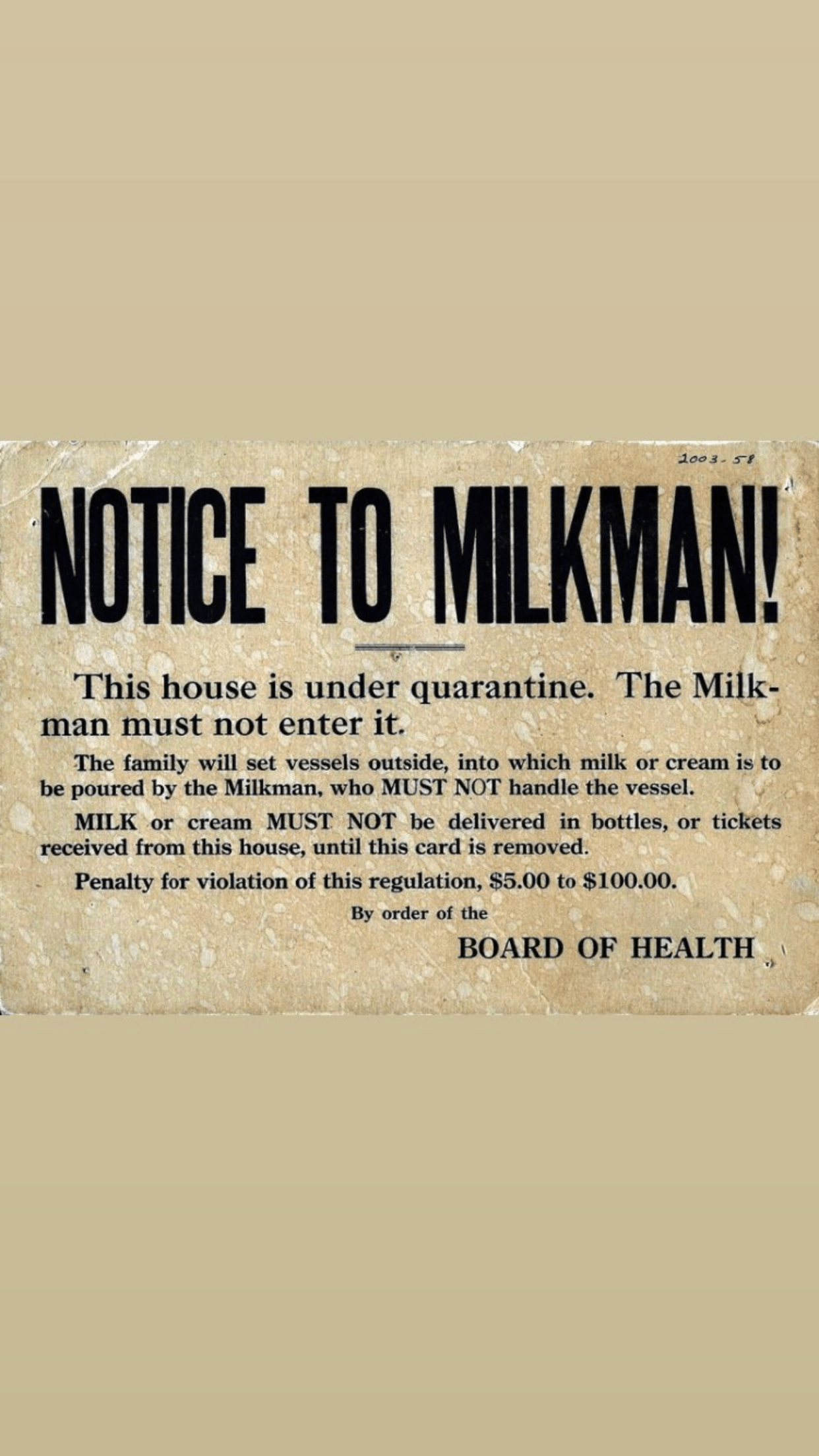xxxxxx
Page 2xxxxxx
Page 3xxx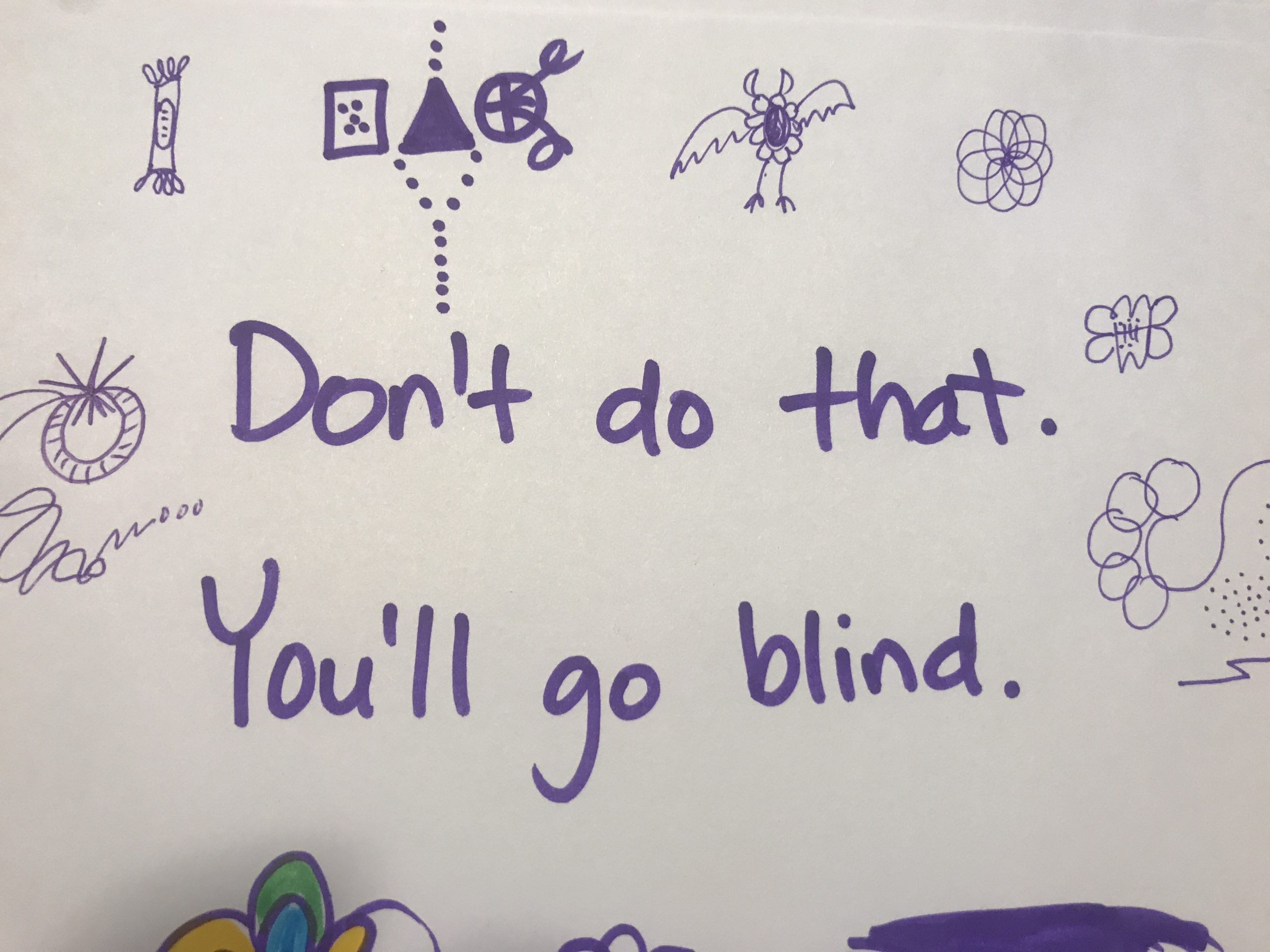x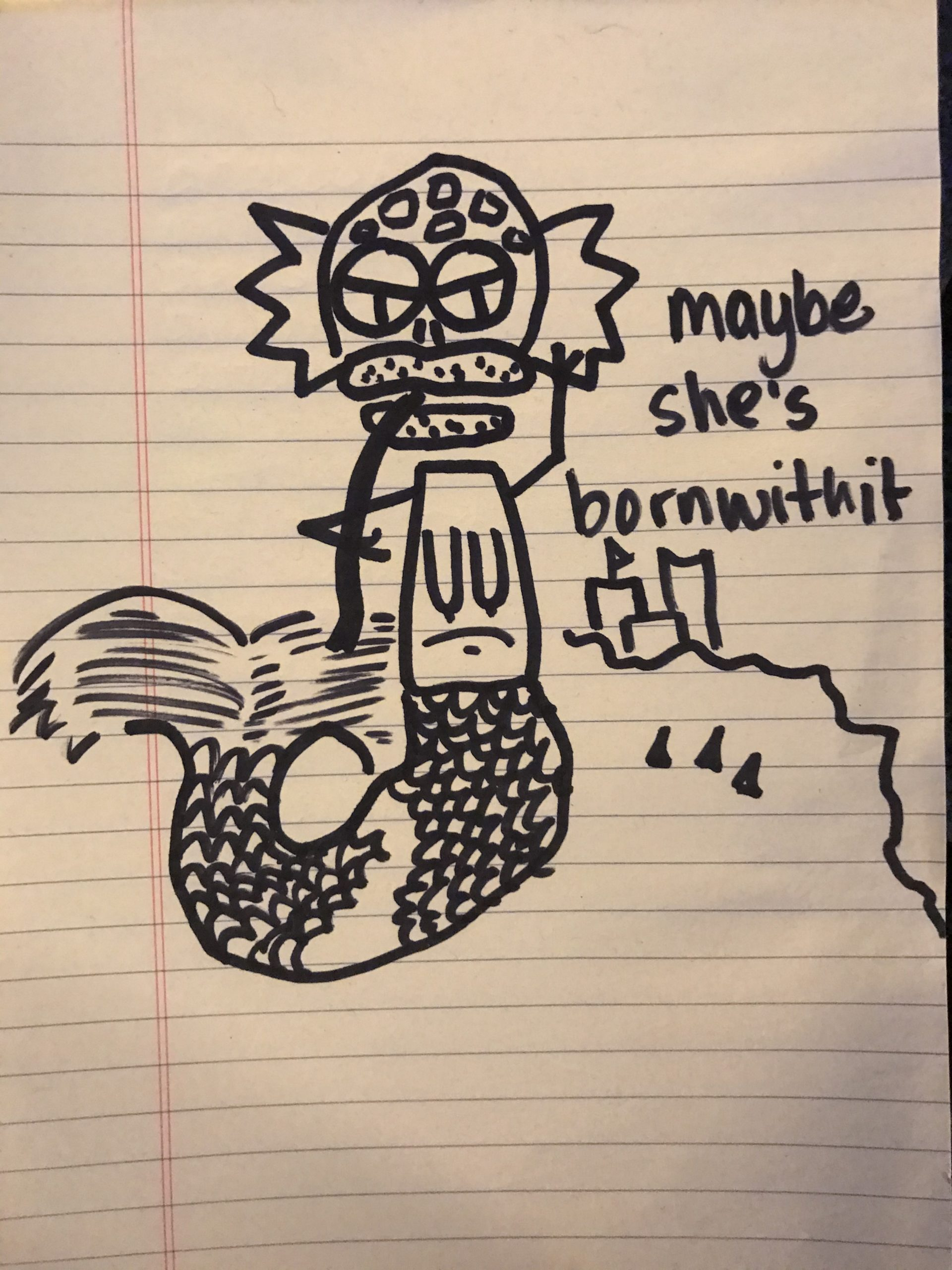xxxx
Page 4xx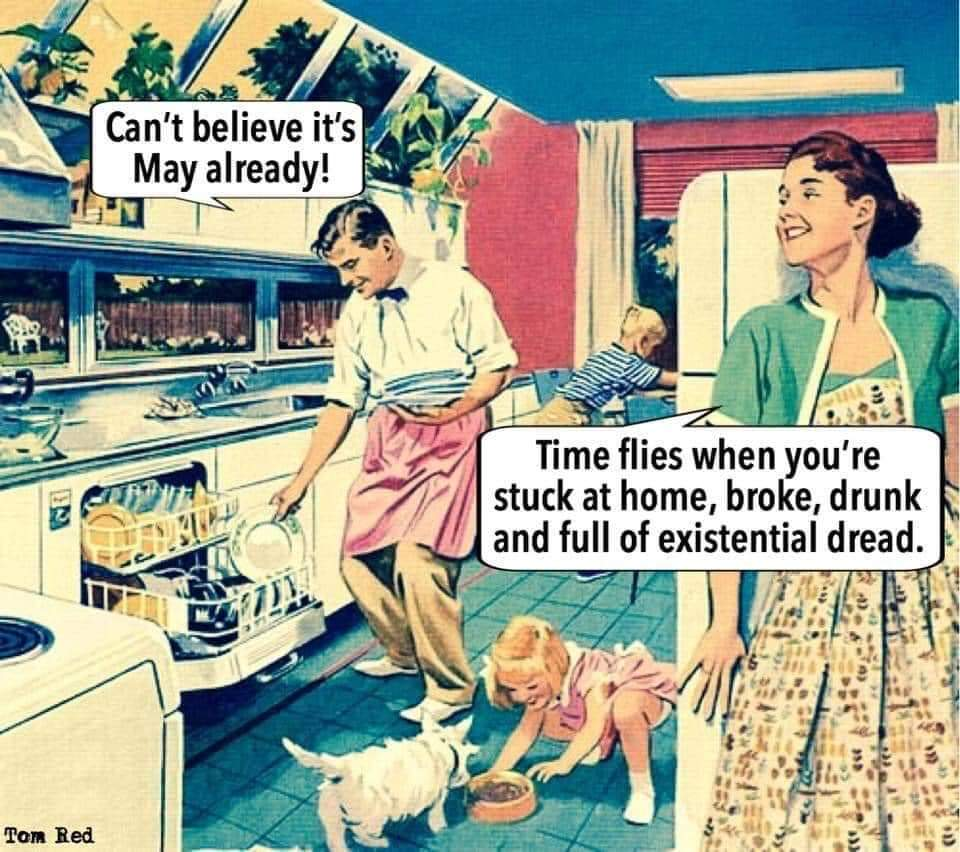xxxx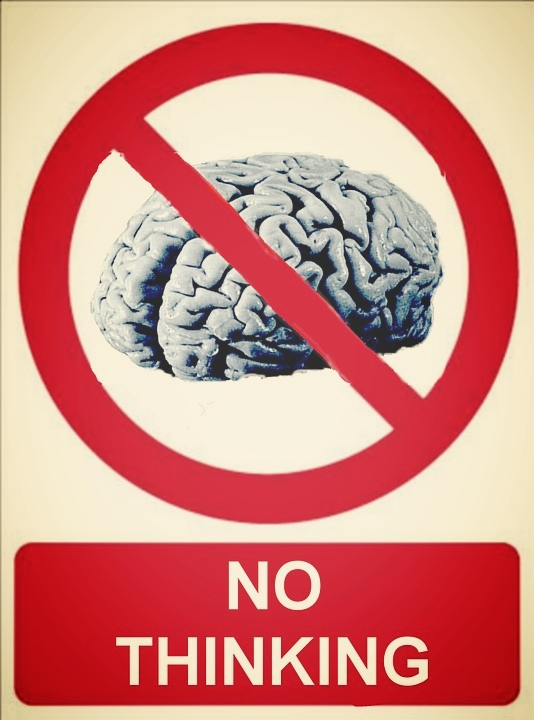x
Page 5xxxxxxxxxx
Page 6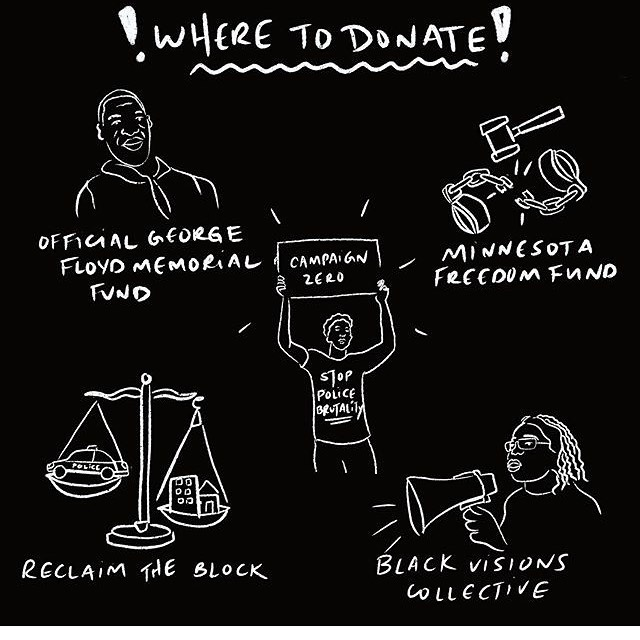xxxx

Page 7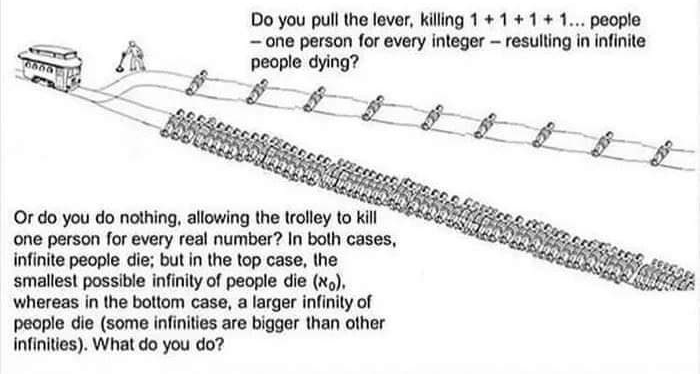xx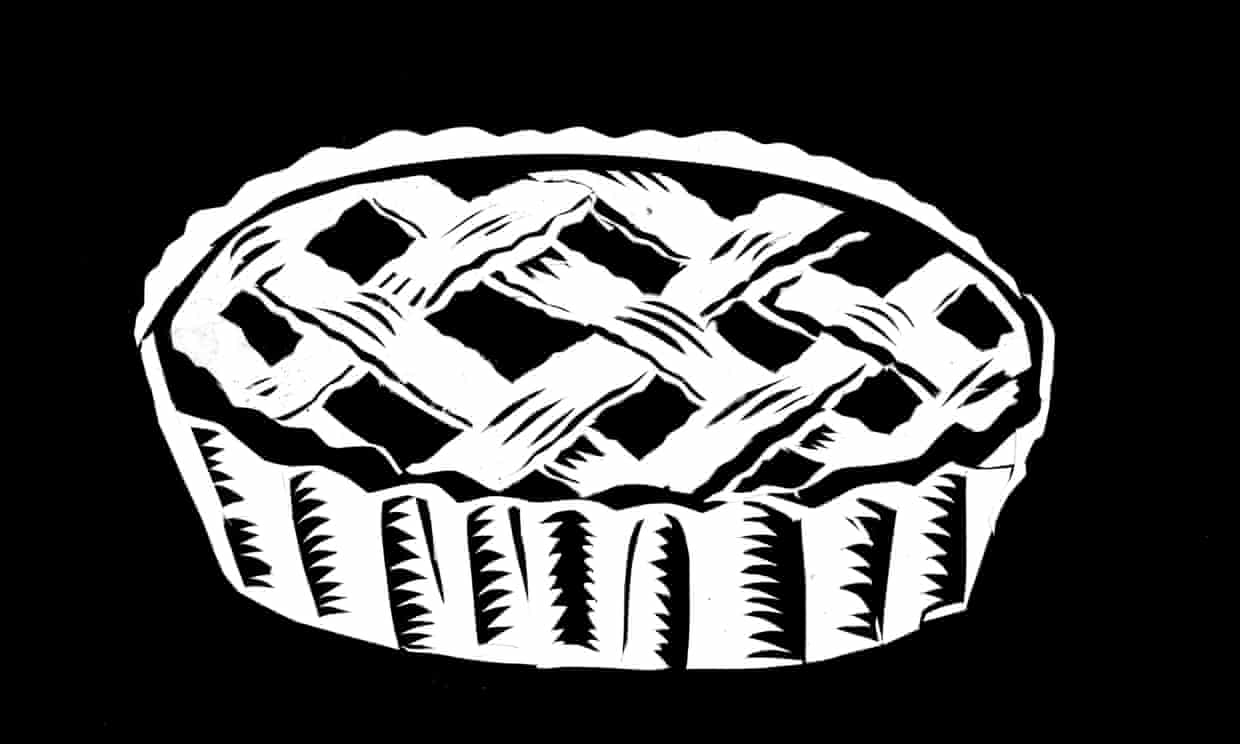xx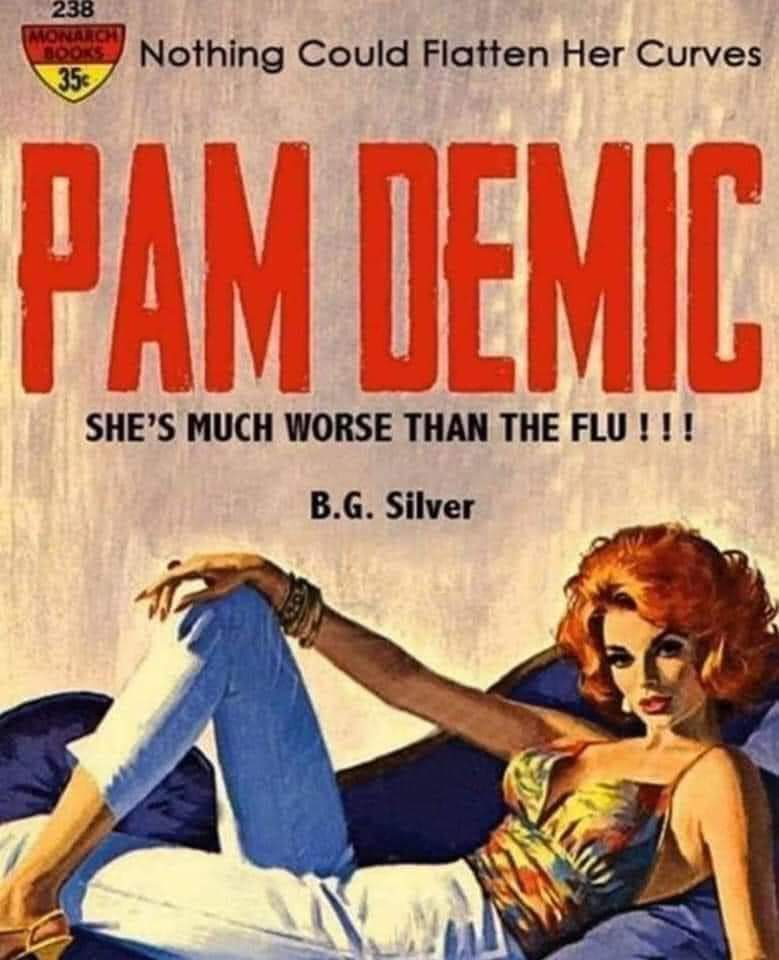xxxx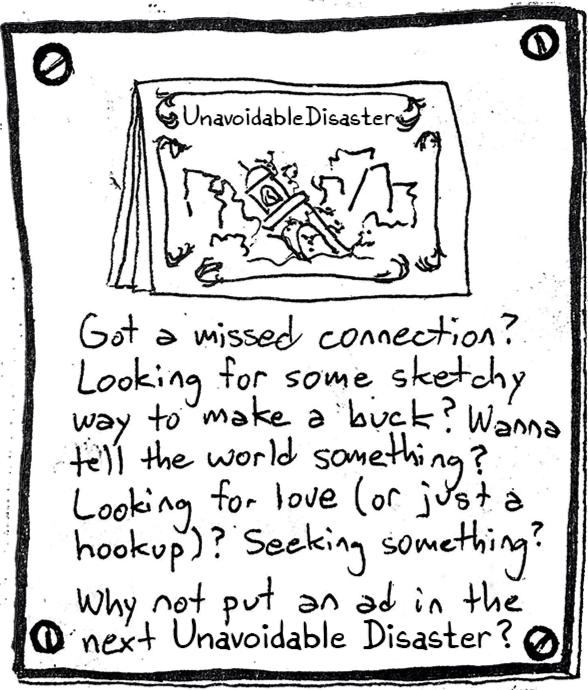xx
Page 8